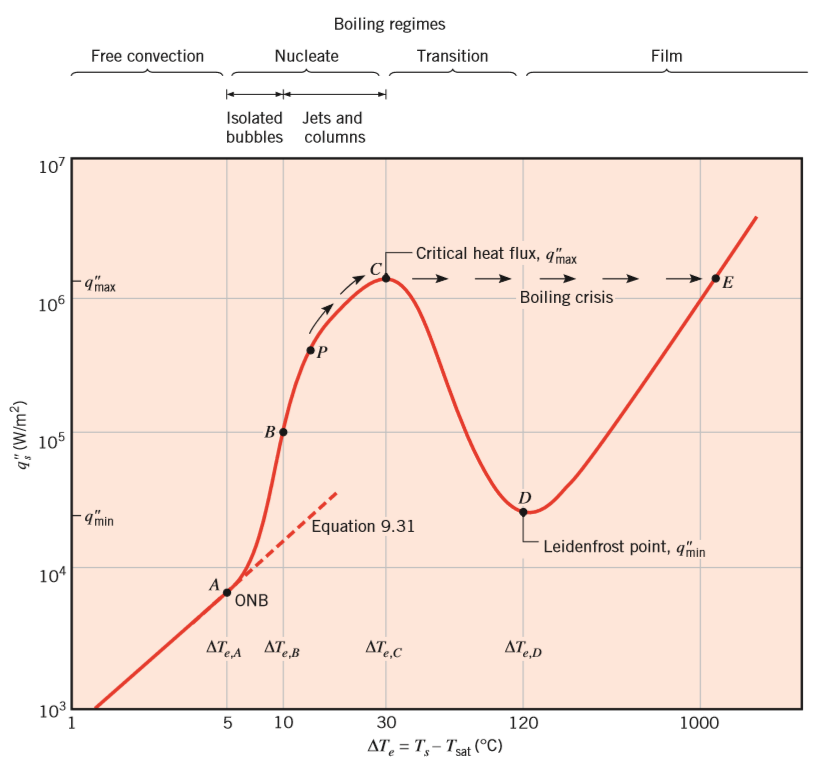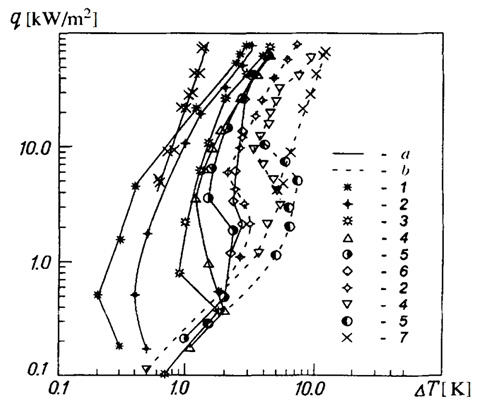# Boiling regime

Nukiyama was the ﬁrst to identify different regimes of pool boiling, representing them in a curve of heat flux densities $Q$ vs the excess temperature $\Delta T_e=T{wall}-T_{sat}$. Although Nukiyama studied pool boiling of water at atmospheric pressure, similar trends characterize the behaviour of other ﬂuids. The shape of the boiling curve and its evolution under different system conditions are a result of different boiling regimes displayed in Figure 2 (Incropera et al. 2011 ).Figure 2: Typical boiling curve for water at 1 atm: surface heat ﬂux as a function of excess temperature (according to Incropera et al. 2011)

Critical heat ﬂux

The critical heat ﬂux represents an important point on the boiling curve. Kutateladze (1948)  and Zuber (1958)  obtained an expression of this value, independent of the surface material and weakly dependent of the geometry, which can be approximated as

$q_{max}=Ch_{fg}\rho_v [\frac{\sigma g(\rho_l-\rho_v)}{\rho_v^2}]^{1/4}$   (1)

For horizontal cylinders, spheres and large finite surfaces, the leading constant C is approximately equal to 0.131 (the Zuber constant). This equation applies when the characteristic length is large relative to the bubble diameter .

The value of critical heat flux of propane, under the conditions used in this project, is $q_{max}=423.32kW/m^2$ . Therefore, for the range of heat fluxes considered, the critical value is not reached and so the first two regimes can be observed: natural convection and nucleate boiling.

Pool boiling curve for propane

The pool boiling curve for propane under different pressure can be seen in the figure below (A. S. Zhuravlev, 2000 ). Curve number 5 in dashed line was considered as reference for comparison, since it represents the curve for propane in saturation conditions at P=474kPa, on a smooth surface. The free convection regime is observed in a wide range of heat flux densities, up to 5 kW/m2 and beyond this value, the nucleate boiling regime takes place, with a transition regime between 2kW/m2 and 5kW/m2.Figure 3: Curves of pool boiling of propane a) specimen with a porous coating, b) with a smooth surface;
1) P/Pcr=0.324; 2) 0.254; 3) 0.197; 4) 0.15; 5) 0.11; 6) 0.081; 7) 0.003. (Acording to A. S. Zhuravlev, 2000 )